We’d like to understand how you use our websites in order to improve them. Register your interest.

# On decay properties of solutions for degenerate Kirchhoff equations with strong damping and source terms

## Abstract

We investigate the degenerate Kirchhoff equations with strong damping and source terms of the form

$ε u t t − ∥ ∇ u ∥ 2 2 γ Δu−Δ u t =f(u),$

in a bounded domain. We obtain the optimal decay rate for $∥ ∇ u t ∥ 2 2$ by deriving its decay estimate from below, provided that either ε is suitably small or the initial data satisfy the proper smallness assumption. The key ingredient in the proof is based on the work of Ono (J. Math. Anal. Appl. 381(1):229-239, 2011), with necessary modification imposed by our problem.

MSC:35L70, 35L80.

## 1 Introduction

In this paper, we consider the initial boundary value problem for the following degenerate Kirchhoff equations with strong damping and source terms: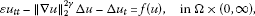(1.1)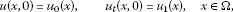(1.2)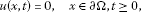(1.3)

where Ω is a bounded domain in $R N$ ($N≥1$) with a smooth boundary Ω. Here, $ε>0$ and $γ>0$ are positive constants and $f(u)$ is a nonlinear term like $|u | p − 1 u$, $p>1$.

In the case $N=1$, the nonlinear vibrations of the elastic string are written in the form:

$ρh ∂ 2 u ∂ t 2 = { p 0 + E h 2 L ∫ 0 L ( ∂ u ∂ x ) 2 d x } ∂ 2 u ∂ x 2 +f,$
(1.4)

for $0, $t≥0$; where u is the lateral deflection, x the space coordinate, t the time, E the Young modulus, ρ the mass density, h the cross section area, L the length, $p 0$ the initial axial tension and f the external force. The equation was first introduced by Kirchhoff  in the study of stretched strings and plates, and is called the wave equation of Kirchhoff type after his name. Moreover, it is said that (1.4) is degenerate if $p 0 =0$ and nondegenerate if $p 0 >0$.

A number of results on the solutions to problem (1.1)-(1.3) have been established by many authors. For example, Hosoya and Yamada  studied the following equation:

$u t t −M ( ∥ ∇ u ∥ 2 2 ) Δu+δ u t =0,$
(1.5)

with $M(r)≥ M 0 >0$ (the nondegenerate case). They proved the global existence of a unique solution under the small data condition in $H 2 (Ω)∩ H 0 1 (Ω)× H 0 1 (Ω)$. Similarly, in the degenerate case ($M(r)= r γ$), Nishihara and Yamada  obtained the global existence of solutions for small initial data in $H 2 (Ω)∩ H 0 1 (Ω)× H 0 1 (Ω)$.

Concerning decay properties of solutions, Nakao  has derived decay estimates from above of solutions when $f(u)=0$ in (1.1). Later, Nishihara  established a decay estimate from below of the potential of solutions to problem (1.1) without imposing $f(u)$. Nishihara and Ono  studied detailed cases of nondegenerate type and degenerate type to problem (1.1)-(1.3). They proved the existence and uniqueness of a global solution for initial data $( u 0 , u 1 )∈ H 2 (Ω)∩ H 0 1 (Ω)× L 2 (Ω)$ and the polynomial decay of the solution. Recently, in the absence of $f(u)$ in (1.1), Ono  proved the optimal decay estimate for $∥ ∇ u ∥ 2 2$. And the decay property of the norm $∥ Δ u ∥ 2 2$ for $t≥0$ was also given in that paper.

Motivated by these works, in this paper, we intend to give the optimal decay estimate for $∥ ∇ u t ∥ 2$ to problem (1.1)-(1.3). In this way, we can extend the result in  where the author considered (1.1) with $γ≥1$; and we also improve the result of  in the presence of a nonlinear source term. We followed the technique skill introduced in  with the concept of a stable set in $H 2 (Ω)∩ H 0 1 (Ω)$ to derive the optimal decay rate of $∥ ∇ u t ∥ 2$. The content of this paper is organized as follows. In Section 2, we give some lemmas which will be used later. In Section 3, we derive the global solution and its decay properties with $γ>0$.

## 2 Preliminary results

In this section, we shall give some lemmas which will be used throughout this work. We denote by $∥ ⋅ ∥ p$ the $L p$ norm over Ω.

Lemma 2.1 (The Sobolev-Poincaré inequality)

If$2≤p≤ 2 N N − 2$ ($2≤p<∞$if$N=1,2$), then

holds with some positive constant$B 1$.

Lemma 2.2 ([4, 9])

Let $ϕ(t)$ be a nonincreasing and nonnegative function on $[0,∞)$ such that

$ϕ ( t ) 1 + r ≤ ω 0 ( ϕ ( t ) − ϕ ( t + 1 ) )$

with certain constants$ω 0 ≥0$and$r>0$. Then, the function$ϕ(t)$satisfies

$ϕ(t)≤ ( ϕ ( 0 ) − r + ω 0 − 1 r [ t − 1 ] + ) − 1 r$

for$t≥0$, where we put$[ a ] + =max{0,a}$and$1 [ a ] + =∞$if$[ a ] + =0$.

Now, we state the local existence for problem (1.1)-(1.3) which can be established by the arguments of .

Theorem 2.3 Suppose that$( u 0 , u 1 )∈ H 2 (Ω)∩ H 0 1 (Ω)× L 2 (Ω)$, then there exists a unique solution u of problem (1.1)-(1.3) satisfying

$u ( t ) ∈ C ( [ 0 , T ) ; H 2 ( Ω ) ∩ H 0 1 ( Ω ) ) , u t ( t ) ∈ C ( [ 0 , T ) ; L 2 ( Ω ) ) ∩ L 2 ( 0 , T ; H 0 1 ( Ω ) ) .$

Moreover, at least one of the following statements holds true:

1. (i)

$T=∞$,

2. (ii)

$∥ u t ∥ 2 2 + ∥ Δ u ∥ 2 2 →∞$, as $t→ T −$.

## 3 Decay properties

In this section, we shall show the decay properties of solutions u to problem (1.1)-(1.3). For this purpose, we define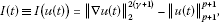(3.1)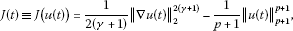(3.2)

and the energy function

$E(t)= ε 2 ∥ u t ∥ 2 2 +J(t),$
(3.3)

for $u(t)∈ H 0 1 (Ω)$, $t≥0$. Then, multiplying (1.1) by $u t$ and integrating it over Ω, we see that

$E ′ (t)=− ∥ ∇ u t ∥ 2 2 .$
(3.4)

Lemma 3.1 Let$( u 0 , u 1 )∈ H 2 (Ω)∩ H 0 1 (Ω)× L 2 (Ω)$. Suppose that p and γ satisfy

(3.5)

If $I(0)>0$ and

$l= B 1 p + 1 ( 2 ( p + 1 ) ( γ + 1 ) p − 2 γ − 1 E ( 0 ) ) p − 2 γ − 1 2 ( γ + 1 ) <1,$
(3.6)

then$I(t)>0$, for$t∈[0,T]$.

Proof Since $I(0)>0$, then there exists $t max such that

$I(t)≥0,t∈[0, t max ],$

which gives

$J(t)= p − 2 γ − 1 2 ( p + 1 ) ( γ + 1 ) ∥ ∇ u ( t ) ∥ 2 2 ( γ + 1 ) + 1 p + 1 I(t)≥ p − 2 γ − 1 2 ( p + 1 ) ( γ + 1 ) ∥ ∇ u ( t ) ∥ 2 2 ( γ + 1 ) .$
(3.7)

Thus, from (3.3) and $E(t)$ being nonincreasing by (3.4), we have that

(3.8)

And so, exploiting Lemma 2.1, (3.8) and (3.6), we obtain

(3.9)

Therefore,

By repeating this procedure and using the fact that

$lim t → t max B 1 p + 1 ( 2 ( p + 1 ) ( γ + 1 ) p − 2 γ − 1 E ( t ) ) p − 2 γ − 1 2 ( γ + 1 ) ≤l<1,$

we can take $t max =T$. □

Lemma 3.2 Let u satisfy the assumptions of Lemma  3.1. Then there exists$0<η<1$such that

(3.10)

with$η=1−l$.

Proof From (3.9), we get

Let $η=1−l$, then we have the inequality (3.10). □

Theorem 3.3 Suppose that$( u 0 , u 1 )∈ H 2 (Ω)∩ H 0 1 (Ω)× L 2 (Ω)$, $I(0)>0$, (3.5) and (3.6) hold. Then there exists a unique global solution u of problem (1.1)-(1.3) satisfying

$u ( t ) ∈ C ( [ 0 , ∞ ) ; H 2 ( Ω ) ∩ H 0 1 ( Ω ) ) ∩ L ∞ ( 0 , ∞ ; H 2 ( Ω ) ∩ H 0 1 ( Ω ) ) , u t ( t ) ∈ C ( [ 0 , ∞ ) ; L 2 ( Ω ) ) ∩ L 2 ( 0 , ∞ ; H 0 1 ( Ω ) ) ∩ L ∞ ( 0 , ∞ ; L 2 ( Ω ) ) .$

Furthermore, we have the following decay estimates:

(3.11)

where α and β are some positive constants given by (3.15).

Proof First, for $T=∞$, we can obtain the result by following the arguments as in , so we omit the proof. Next, we will show the decay estimate. For $t≥0$, integrating (3.4) over $[t,t+1]$, we have

$∫ t t + 1 ∥ ∇ u t ( s ) ∥ 2 2 ds=E(t)−E(t+1)≡D ( t ) 2 .$
(3.12)

Then there exist $t 1 ∈[t,t+ 1 4 ]$ and $t 2 ∈[t+ 3 4 ,t+1]$ such that

$∥ ∇ u t ( t i ) ∥ 2 2 ≤4D ( t ) 2 ,i=1,2.$
(3.13)

Multiplying (1.1) by u, integrating it over $Ω×[ t 1 , t 2 ]$, using integration by parts and Lemma 2.1, we get

$∫ t 1 t 2 ( ∥ ∇ u ( s ) ∥ 2 2 ( γ + 1 ) − ∥ u ( s ) ∥ p + 1 p + 1 ) d s ≤ ε B 1 2 ∫ t t + 1 ∥ ∇ u t ∥ 2 2 d s + ε B 1 2 ∑ i = 1 2 ∥ ∇ u t ( t i ) ∥ 2 ∥ ∇ u ( t i ) ∥ 2 + ∫ t t + 1 ∥ ∇ u t ∥ 2 ∥ ∇ u ∥ 2 d s .$

Hence, from the definition of $I(t)$ by (3.1), using (3.12), (3.13) and (3.8), we obtain

$∫ t 1 t 2 I(s)ds≤ε B 1 2 D ( t ) 2 + ( 4 ε B 1 2 + 1 ) D(t) ( 2 ( p + 1 ) ( γ + 1 ) p − 2 γ − 1 E ( t ) ) 1 2 ( γ + 1 ) .$
(3.14)

On the other hand, integrating (3.4) over $[t, t 2 ]$, noting that $E( t 2 )≤2 ∫ t 1 t 2 E(t)dt$ due to $t 2 − t 1 ≥ 1 2$, and using (3.3) and (3.7), we have that

$E ( t ) = E ( t 2 ) + ∫ t t 2 ∥ ∇ u t ( s ) ∥ 2 2 d s ≤ 2 ∫ t 1 t 2 E ( s ) d s + ∫ t t 2 ∥ ∇ u t ( s ) ∥ 2 2 d s ≤ ( 1 + ε B 1 2 ) ∫ t t 2 ∥ ∇ u t ( s ) ∥ 2 2 d s + p − 2 γ − 1 ( p + 1 ) ( γ + 1 ) ∫ t t 2 ∥ ∇ u ( s ) ∥ 2 2 ( γ + 1 ) d s + 2 ( p + 1 ) ∫ t t 2 I ( s ) d s .$

We then use the fact that $∥ ∇ u ( t ) ∥ 2 2 ( γ + 1 ) ≤ 1 1 − l I(t)$ by (3.10), (3.12) and (3.14) to obtain

$E ( t ) ≤ ( 1 + ε B 1 2 ) D ( t ) 2 + c 1 ∫ t t 2 I ( t ) d t ≤ α D ( t ) 2 + β D ( t ) E ( t ) 1 2 ( γ + 1 ) ,$
(3.15)

where

$α= ( 1 + ε B 1 2 + c 1 ε B 1 2 ) andβ= c 1 ( 4 ε B 1 2 + 1 ) ( 2 ( p + 1 ) ( γ + 1 ) p − 2 γ − 1 ) 1 2 ( γ + 1 )$
(3.16)

with

$c 1 = p − 2 γ − 1 ( p + 1 ) ( γ + 1 ) ( 1 − l ) + 2 ( p + 1 ) .$

Moreover, from (3.12) and $E(t)$ being nonincreasing by (3.4), we note that

$D(t)≤ E 1 2 (t)≤E ( 0 ) γ 2 ( γ + 1 ) E ( t ) 1 2 ( γ + 1 ) .$

Thus, by Young’s inequality, (3.15) becomes

$E ( t ) ≤ ( α E ( 0 ) γ 2 ( γ + 1 ) + β ) D ( t ) E ( t ) 1 2 ( γ + 1 ) ≤ 2 γ + 1 2 ( γ + 1 ) [ ( α E ( 0 ) γ 2 ( γ + 1 ) + β ) D ( t ) ] 2 ( γ + 1 ) 2 γ + 1 + 1 2 ( γ + 1 ) E ( t ) .$

This implies that

$E ( t ) 1 + γ γ + 1 ≤ ( α E ( 0 ) γ 2 ( γ + 1 ) + β ) 2 D 2 ( t ) = ( α E ( 0 ) γ 2 ( γ + 1 ) + β ) 2 ( E ( t ) − E ( t + 1 ) ) .$

Therefore, applying Lemma 2.2, we conclude that

□

Remark (i) Based on the estimate (3.11), we have further the following estimates:

(3.17)

where $c 2$ and $c 3$ are some positive constants.

1. (ii)

If $ω> γ γ + 1$, then

$∫ 0 t E ( s ) ω ds≤ k 1 E ( 0 ) ω − γ γ + 1 ,$
(3.18)

with $k 1 = ( γ + 1 ) 2 ω − γ ( ( γ + 1 ) ω − γ ) ( γ + 1 ) ( α E ( 0 ) γ 2 ( γ + 1 ) + β ) 2$. Indeed, by (3.11), we see that

$∫ 0 t E ( s ) ω d s ≤ ∫ 0 t ( E ( 0 ) − γ γ + 1 + γ + 1 γ ( α E ( 0 ) γ 2 ( γ + 1 ) + β ) − 2 [ s − 1 ] + ) − ( γ + 1 ) ω γ d s = E ( 0 ) ω + ∫ 1 t ( E ( 0 ) − γ γ + 1 + γ + 1 γ ( α E ( 0 ) γ 2 ( γ + 1 ) + β ) − 2 ( s − 1 ) ) − ( γ + 1 ) ω γ d s .$

A direct computation yields the result.

Next, we will improve the decay rate for $∥ u t ∥ 2 2$ given by (3.17).

Proposition 3.4 If$( u 0 , u 1 )∈ H 2 (Ω)∩ H 0 1 (Ω)× L 2 (Ω)$, then the solution u of problem (1.1)-(1.3) satisfies

(3.19)

where$c 4$and$c 5$are some positive constants.

Proof Multiplying (1.1) by $u t$ and integrating it over Ω, we have

$ε 2 d d t ∥ u t ∥ 2 2 + ∥ ∇ u t ∥ 2 2 =− ∥ ∇ u ∥ 2 2 γ ∫ Ω ∇u∇ u t dx+ ∫ Ω |u | p − 1 u u t dx.$
(3.20)

We now estimate the right-hand side of (3.20). Employing Hölder’s inequality and Young’s inequality, the first term gives

$∥ ∇ u ∥ 2 2 γ ∫ Ω ∇ u ∇ u t d x ≤ ∥ ∇ u ∥ 2 2 γ + 1 ∥ ∇ u t ∥ 2 ≤ ∥ ∇ u ∥ 2 2 ( 2 γ + 1 ) + 1 4 ∥ ∇ u t ∥ 2 2 .$

As for the second term, using Hölder’s inequality, Lemma 2.1, Young’s inequality and (3.8) yields

$| ∫ Ω | u | p − 1 u u t d x | ≤ ∥ u ∥ p + 1 p ∥ u t ∥ p + 1 ≤ B 1 p + 1 ∥ ∇ u ∥ 2 p ∥ ∇ u t ∥ 2 ≤ B 1 2 ( p + 1 ) ∥ ∇ u ∥ 2 2 p + 1 4 ∥ ∇ u t ∥ 2 2 ≤ B 1 2 ( p + 1 ) ( 2 ( p + 1 ) ( γ + 1 ) p − 2 γ − 1 E ( 0 ) ) p − 2 γ − 1 γ + 1 ∥ ∇ u ∥ 2 2 ( 2 γ + 1 ) + 1 4 ∥ ∇ u t ∥ 2 2 .$

Combining these estimates and using (3.17), we arrive at

$ε d d t ∥ u t ∥ 2 2 + ∥ ∇ u t ∥ 2 2 ≤ c 6 ∥ ∇ u ∥ 2 2 ( 2 γ + 1 ) ≤ c 7 ( 1 + t ) − 2 − 1 γ ,$

where $c 6 =2(1+ B 1 2 ( p + 1 ) ( 2 ( p + 1 ) ( γ + 1 ) p − 2 γ − 1 E ( 0 ) ) p − 2 γ − 1 γ + 1 )$ and $c 7 = c 6 c 2 2 γ + 1$. Then, from Lemma 2.1, we have

$ε d d t ∥ u t ∥ 2 2 + B 1 − 1 ∥ u t ∥ 2 2 ≤ c 7 ( 1 + t ) − 2 − 1 γ .$

Therefore, the desired estimate (3.19) is obtained. □

In order to obtain the decay estimates for problem (1.1)-(1.3), we need the function $H(t)$ and equality (3.22) as in . Define

(3.21)

Multiplying (1.1) by $2 u t ∥ ∇ u ∥ 2 2 ( γ + k )$ with $k≥0$ and integrating it over Ω, we have the following equality: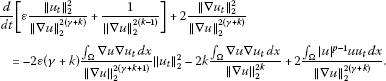(3.22)

Proposition 3.5 Let$( u 0 , u 1 )∈ H 2 (Ω)∩ H 0 1 (Ω)× L 2 (Ω)$, $I(0)>0$, (3.5)-(3.6) hold and$∥ ∇ u 0 ∥ 2 >0$. Suppose that$∥ ∇ u ( t ) ∥ 2 >0$for$0≤t< T 1$and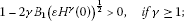(3.23)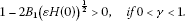(3.24)

Then it holds that, for$0≤t< T 1$,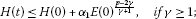(3.25)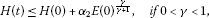(3.26)

where constants$α 1$and$α 2$are given by (3.31) and (3.36).

Proof (i) In the case $γ≥1$, we observe from (3.21) that

$ε ∥ u t ∥ 2 ∥ ∇ u ∥ 2 = ε 1 2 ( ε ∥ u t ∥ 2 2 ∥ ∇ u ∥ 2 2 γ ∥ ∇ u ∥ 2 2 γ − 2 ) 1 2 ≤ ( ε H γ ( t ) ) 1 2 .$
(3.27)

Using (3.22) with $k=0$ yields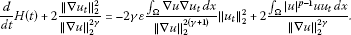(3.28)

Now, we estimate the right-hand side of (3.28). So, thanks to Hölder’s inequality, Lemma 2.1, (3.8) and Young’s inequality, we see that

$2γε ∫ Ω ∇ u ∇ u t d x ∥ ∇ u ∥ 2 2 ( γ + 1 ) ∥ u t ∥ 2 2 ≤2γ B 1 ε ∥ u t ∥ 2 ∥ ∇ u ∥ 2 ∥ ∇ u t ∥ 2 2 ∥ ∇ u ∥ 2 2 γ ,$

and

$2 ∫ Ω | u | p − 1 u u t d x ∥ ∇ u ∥ 2 2 γ ≤ 2 B 1 p + 1 ∥ ∇ u ∥ 2 p − γ ∥ ∇ u t ∥ 2 ∥ ∇ u ∥ 2 γ ≤ 2 B 1 p + 1 ( 2 ( p + 1 ) ( γ + 1 ) p − 2 γ − 1 E ( t ) ) p − γ 2 ( γ + 1 ) ∥ ∇ u t ∥ 2 ∥ ∇ u ∥ 2 γ ≤ B 1 2 ( p + 1 ) ( 2 ( p + 1 ) ( γ + 1 ) p − 2 γ − 1 E ( t ) ) p − γ γ + 1 + ∥ ∇ u t ∥ 2 2 ∥ ∇ u ∥ 2 2 γ .$

Taking into account these two estimates in (3.28) and using $ε ∥ u t ∥ 2 ∥ ∇ u ∥ 2 ≤ ( ε H γ ( t ) ) 1 2$ by (3.27), we obtain

$d d t H(t)+ ( 1 − 2 γ B 1 ( ε H γ ( t ) ) 1 2 ) ∥ ∇ u t ∥ 2 2 ∥ ∇ u ∥ 2 2 γ ≤ B 1 2 ( p + 1 ) ( 2 ( p + 1 ) ( γ + 1 ) p − 2 γ − 1 E ( t ) ) p − γ γ + 1 .$

If $1−2γ B 1 ( ε H γ ( 0 ) ) 1 2 >0$, then there exists $T 2$ such that $0< T 2 ≤ T 1$ and

(3.29)

Hence

$d d t H(t)≤ B 1 2 ( p + 1 ) ( 2 ( p + 1 ) ( γ + 1 ) p − 2 γ − 1 E ( t ) ) p − γ γ + 1 ,$
(3.30)

for $0≤t≤ T 2$. Applying (3.18) with $ω= p − γ γ + 1$, we derive that

$H(t)≤H(0)+ α 1 E ( 0 ) p − 2 γ γ + 1 ,$
(3.31)

with

$α 1 = B 1 2 ( p + 1 ) ( 2 ( p + 1 ) ( γ + 1 ) p − 2 γ − 1 ) p − γ γ + 1 ( γ + 1 ) ( p − γ ) − γ ( p − 2 γ ) ( γ + 1 ) ( α E ( 0 ) γ 2 ( γ + 1 ) + β ) 2 .$

Therefore, we see that (3.29)-(3.31) hold true for $0≤t< T 1$, which gives the estimate (3.25) for $γ≥1$.

1. (ii)

In the case $0<γ<1$, we note from (3.21) that

$ε ∥ u t ∥ 2 ∥ ∇ u ∥ 2 ≤ ( ε H ( t ) ) 1 2 .$
(3.32)

From (3.22) with $k=1−γ$, we have

$d d t H ( t ) + 2 ∥ ∇ u t ∥ 2 2 ∥ ∇ u ∥ 2 2 = − 2 ε ∫ Ω ∇ u ∇ u t d x ∥ ∇ u ∥ 2 4 ∥ u t ∥ 2 2 − 2 ( 1 − γ ) ∫ Ω ∇ u ∇ u t d x ∥ ∇ u ∥ 2 2 ( 1 − γ ) + 2 ∫ Ω | u | p − 1 u u t d x ∥ ∇ u ∥ 2 2 .$
(3.33)

Similar to those estimates, as in the case for $γ≥1$, the right-hand side of (3.33) can be estimated as follows: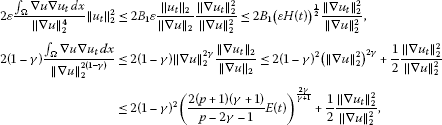and

$2 ∫ Ω | u | p − 1 u u t d x ∥ ∇ u ∥ 2 2 ≤2 B 1 2 ( p + 1 ) ( 2 ( p + 1 ) ( γ + 1 ) p − 2 γ − 1 E ( t ) ) p − 1 γ + 1 + 1 2 ∥ ∇ u t ∥ 2 2 ∥ ∇ u ∥ 2 2 .$

Combining these estimates, (3.33) becomes

$d d t H ( t ) + ( 1 − 2 B 1 ( ε H ( t ) ) 1 2 ) ∥ ∇ u t ∥ 2 2 ∥ ∇ u ∥ 2 2 ≤ 2 ( 1 − γ ) 2 ( 2 ( p + 1 ) ( γ + 1 ) p − 2 γ − 1 ) 2 γ γ + 1 E ( t ) 2 γ γ + 1 + 2 B 1 2 ( p + 1 ) ( 2 ( p + 1 ) ( γ + 1 ) p − 2 γ − 1 ) p − 1 γ + 1 E ( t ) p − 1 γ + 1 = c 8 E ( 0 ) 2 γ γ + 1 ( E ( t ) E ( 0 ) ) 2 γ γ + 1 + c 9 E ( 0 ) p − 1 γ + 1 ( E ( t ) E ( 0 ) ) p − 1 γ + 1 ≤ c 10 ( E ( t ) ) 2 γ γ + 1 ,$

where we have used the fact that $0 and $p>2γ+1$ on the last inequality with $c 8 =2 ( 1 − γ ) 2 ( 2 ( p + 1 ) ( γ + 1 ) p − 2 γ − 1 ) 2 γ γ + 1$, $c 9 =2 B 1 2 ( p + 1 ) ( 2 ( p + 1 ) ( γ + 1 ) p − 2 γ − 1 ) p − 1 γ + 1$ and $c 10 = c 8 + c 9 E ( 0 ) p − 2 γ − 1 γ + 1$.

If $1−2 B 1 ( ε H ( 0 ) ) 1 2 >0$, then there exists $T 3$ such that $0< T 3 ≤ T 1$ and

(3.34)

Thus

$d d t H(t)≤ c 10 ( E ( t ) ) 2 γ γ + 1 ,$
(3.35)

for $0≤t≤ T 3$. Employing (3.18) with $ω= 2 γ γ + 1$ gives

(3.36)

with

$α 2 = ( 2 γ + 1 ) c 10 γ + 1 ( α E ( 0 ) γ 2 ( γ + 1 ) + β ) 2 .$

Therefore, we see that (3.34)-(3.36) hold true for $0≤t< T 1$, which gives the estimate (3.26) for $0<γ<1$. □

Proposition 3.6 Under the same assumptions as in Proposition  3.5, assume further that the initial data satisfies

(3.37)

Then it holds that

(3.38)

where$α 3$is a positive constant.

Proof (i) In the case $γ≥1$, we obtain from (3.22) with $k=2$ that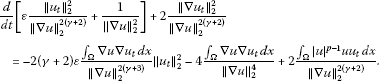(3.39)

As in deriving the estimates for case (i) of Proposition 3.5, we get the following estimates:

$2 ( γ + 2 ) ε ∫ Ω ∇ u ∇ u t d x ∥ ∇ u ∥ 2 2 ( γ + 3 ) ∥ u t ∥ 2 2 ≤ 2 ( γ + 2 ) B 1 ε ∥ u t ∥ 2 ∥ ∇ u ∥ 2 ∥ ∇ u t ∥ 2 2 ∥ ∇ u ∥ 2 2 ( γ + 2 ) , 4 ∫ Ω ∇ u ∇ u t d x ∥ ∇ u ∥ 2 4 ≤ 1 2 ∥ ∇ u t ∥ 2 2 ∥ ∇ u ∥ 2 2 ( γ + 2 ) + 8 ∥ ∇ u ∥ 2 2 ( γ − 1 ) ,$

and

$2 ∫ Ω | u | p − 1 u u t d x ∥ ∇ u ∥ 2 2 ( γ + 2 ) ≤ 1 2 ∥ ∇ u t ∥ 2 2 ∥ ∇ u ∥ 2 2 ( γ + 2 ) +2 B 1 2 ( p + 1 ) ∥ ∇ u ∥ 2 2 ( p − γ − 2 ) .$

Thus, using the above estimates in (3.39), together with the fact that $ε ∥ u t ∥ 2 ∥ ∇ u ∥ 2 ≤ ( ε H γ ( t ) ) 1 2$ by (3.27) and the estimate (3.25), yield

$d d t [ ε ∥ u t ∥ 2 2 ∥ ∇ u ∥ 2 2 ( γ + 2 ) + 1 ∥ ∇ u ∥ 2 2 ] + ∥ ∇ u t ∥ 2 2 ∥ ∇ u ∥ 2 2 ( γ + 2 ) ≤ 2 ( γ + 2 ) B 1 ( ε H γ ( t ) ) 1 2 ∥ ∇ u t ∥ 2 2 ∥ ∇ u ∥ 2 2 ( γ + 2 ) + 8 ∥ ∇ u ∥ 2 2 ( γ − 1 ) + 2 B 1 2 ( p + 1 ) ∥ ∇ u ∥ 2 2 ( p − γ − 2 ) ≤ 2 ( γ + 2 ) B 1 ( ε ( H ( 0 ) + α 1 E ( 0 ) p − 2 γ γ + 1 ) γ ) 1 2 ∥ ∇ u t ∥ 2 2 ∥ ∇ u ∥ 2 2 ( γ + 2 ) + 8 ∥ ∇ u ∥ 2 2 ( γ − 1 ) + 2 B 1 2 ( p + 1 ) ∥ ∇ u ∥ 2 2 ( p − γ − 2 ) ,$

for $0≤t< T 1$.

If $1−2(γ+2) B 1 ( ε ( H ( 0 ) + α 1 E ( 0 ) p − 2 γ γ + 1 ) γ ) 1 2 >0$, then, using $∥ ∇ u ∥ 2 2 ≤ c 2 ( 1 + t ) − 1 γ$ by (3.17), we see that

$d d t [ ε ∥ u t ∥ 2 2 ∥ ∇ u ∥ 2 2 ( γ + 2 ) + 1 ∥ ∇ u ∥ 2 2 ] ≤ c 11 ( ( 1 + t ) − γ − 1 γ + ( 1 + t ) − p − γ − 2 γ ) ≤ 2 c 11 ( 1 + t ) − λ 1 = 2 c 11 ( 1 + t ) − γ − 1 γ ,$

and

$ε ∥ u t ∥ 2 2 ∥ ∇ u ∥ 2 2 ( γ + 2 ) + 1 ∥ ∇ u ∥ 2 2 ≤ c 12 ( 1 + t ) 1 γ ,$

which implies that

with $λ 1 =min{ γ − 1 γ , p − γ − 2 γ }= γ − 1 γ$ and some positive constants $c i$, $i=11-13$.

1. (ii)

In the case $0<γ<1$, it follows from (3.22) with $k=1+γ$ that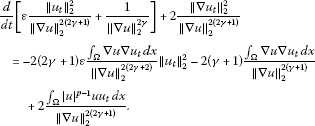(3.40)

Estimate the right-hand side of (3.40) as in the case (ii) of Proposition 3.5 to obtain

$2 ( 2 γ + 1 ) ε ∫ Ω ∇ u ∇ u t d x ∥ ∇ u ∥ 2 2 ( 2 γ + 2 ) ∥ u t ∥ 2 2 ≤ 2 ( 2 γ + 1 ) B 1 ( ε H ( t ) ) 1 2 ∥ ∇ u t ∥ 2 2 ∥ ∇ u ∥ 2 2 ( 2 γ + 1 ) , 2 ( γ + 1 ) ∫ Ω ∇ u ∇ u t d x ∥ ∇ u ∥ 2 2 ( γ + 1 ) ≤ 2 ( γ + 1 ) 2 + 1 2 ∥ ∇ u t ∥ 2 2 ∥ ∇ u ∥ 2 2 ( 2 γ + 1 ) ,$

and

$2 ∫ Ω | u | p − 1 u u t d x ∥ ∇ u ∥ 2 2 ( 2 γ + 1 ) ≤2 B 1 2 ( p + 1 ) ∥ ∇ u ∥ 2 2 ( p − 2 γ − 1 ) + 1 2 ∥ ∇ u t ∥ 2 2 ∥ ∇ u ∥ 2 2 ( 2 γ + 1 ) .$

Back to (3.40), employing these estimates and using (3.26), we deduce that

$d d t [ ε ∥ u t ∥ 2 2 ∥ ∇ u ∥ 2 2 ( 2 γ + 1 ) + 1 ∥ ∇ u ∥ 2 2 γ ] + ∥ ∇ u t ∥ 2 2 ∥ ∇ u ∥ 2 2 ( 2 γ + 1 ) ≤ 2 ( 2 γ + 1 ) B 1 ( ε H ( t ) ) 1 2 ∥ ∇ u t ∥ 2 2 ∥ ∇ u ∥ 2 2 ( 2 γ + 1 ) + 2 ( γ + 1 ) 2 + 2 B 1 2 ( p + 1 ) ( ∥ ∇ u ∥ 2 2 ) ( p − 2 γ − 1 ) ≤ 2 ( 2 γ + 1 ) B 1 ( ε ( H ( 0 ) + α 2 E ( 0 ) γ γ + 1 ) ) 1 2 ∥ ∇ u t ∥ 2 2 ∥ ∇ u ∥ 2 2 ( 2 γ + 1 ) + 2 ( γ + 1 ) 2 + 2 B 1 2 ( p + 1 ) ( ∥ ∇ u ∥ 2 2 ) ( p − 2 γ − 1 )$

for $0≤t< T 1$.

If $1−2(2γ+1) B 1 ( ε ( H ( 0 ) + α 2 E ( 0 ) γ γ + 1 ) ) 1 2 >0$, then, by $∥ ∇ u ∥ 2 2 ≤ c 2 ( 1 + t ) − 1 γ$, we have

$d d t [ ε ∥ u t ∥ 2 2 ∥ ∇ u ∥ 2 2 ( 2 γ + 1 ) + 1 ∥ ∇ u ∥ 2 2 γ ] ≤2 ( γ + 1 ) 2 + c 14 ( 1 + t ) − p − 2 γ − 1 γ ≤ c 15 ,$

and

$ε ∥ u t ∥ 2 2 ∥ ∇ u ∥ 2 2 ( 2 γ + 1 ) + 1 ∥ ∇ u ∥ 2 2 γ ≤ c 16 (1+t),$

for $0≤t< T 1$ with some positive constants $c i$, $i=14-16$. This implies the desired estimate (3.38) for $0<γ<1$. □

Now, we are ready to state and prove our main result.

Theorem 3.7 Let$( u 0 , u 1 )∈ H 2 (Ω)∩ H 0 1 (Ω)× L 2 (Ω)$, $I(0)>0$and (3.5)-(3.6) hold. Then the solution u of problem (1.1)-(1.3) satisfies

(3.41)

Moreover, suppose that the initial data satisfies$u 0 ≠0$and

(3.42)

Then

(3.43)

with some positive constants$α i$, $i=4,5,6$.

Proof Thanks to the decay estimates (3.11) and (3.17), we obtain (3.41). Setting

then, we see that $T 1 >0$ and $∥ ∇ u ( t ) ∥ 2 2 >0$ for $0≤t< T 1$, because of $∥ ∇ u 0 ∥ 2 >0$. If $T 1 <∞$, then it holds that

$∥ ∇ u ( T 1 ) ∥ 2 2 =0.$
(3.44)

However, from $∥ ∇ u ∥ 2 2 ≥ α 3 ( 1 + t ) − 1 γ$ by (3.38), we observe that

$lim t → T 1 ∥ ∇ u ( t ) ∥ 2 2 ≥C ( 1 + T 1 ) − 1 γ >0,$

which contradicts (3.44). Hence, we have $T 1 =∞$ and $∥ ∇ u ( t ) ∥ 2 2 >0$ for all $t≥0$. Therefore, from (3.38), we have

which gives the decay estimate (3.44). □

## References

1. 1.

Kirchhoff G: Vorlesungen űber Mechanik. Teubner, Leipzig; 1883.

2. 2.

Hosoys M, Yamada Y: On some nonlinear wave equations II: global existence and energy decay of solutions. J. Fac. Sci., Univ. Tokyo, Sect. 1A, Math. 1991, 38: 239-250.

3. 3.

Nishihara K, Yamada Y: On global solutions of some degenerate quasilinear hyperbolic equations with dissipative terms. Funkc. Ekvacioj 1990, 33: 151-159.

4. 4.

Nakao M: A difference inequality and its application to nonlinear evolution equations. J. Math. Soc. Jpn. 1978, 30: 747-762. 10.2969/jmsj/03040747

5. 5.

Nishihara K: Decay properties of solutions of some quasilinear hyperbolic equations with strong damping. Nonlinear Anal. 1993, 21: 17-21. 10.1016/0362-546X(93)90174-Q

6. 6.

Nishihara K, Ono K: On a nonlinear degenerate integro-differential equation of hyperbolic type with a strong dissipation. Adv. Math. Sci. Appl. 1995, 5(2):457-476.

7. 7.

Ono K: Global existence and decay properties of solutions for some mildly degenerate nonlinear dissipative Kirchhoff strings. Funkc. Ekvacioj 1997, 40(2):255-270.

8. 8.

Ono K: On global existence, asymptotic stability and blowing up of solutions for some degenerate nonlinear wave equations of Kirchhoff type with strong dissipation. Math. Methods Appl. Sci. 1997, 20: 151-177. 10.1002/(SICI)1099-1476(19970125)20:2<151::AID-MMA851>3.0.CO;2-0

9. 9.

Ono K: On decay properties of solutions for degenerate strongly damped wave equations of Kirchhoff type. J. Math. Anal. Appl. 2011, 381(1):229-239. 10.1016/j.jmaa.2011.03.034

## Acknowledgements

This work was funded by National Taipei University of Technology under the project 101T458-13.

## Author information

Authors

### Corresponding author

Correspondence to Shun-Tang Wu.

### Competing interests

The author declares that they have no competing interests.

## Rights and permissions

Reprints and Permissions

Wu, S. On decay properties of solutions for degenerate Kirchhoff equations with strong damping and source terms. Bound Value Probl 2012, 93 (2012). https://doi.org/10.1186/1687-2770-2012-93